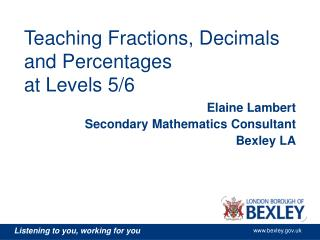DownloadDownload PresentationTeaching Fractions, Decimals and Percentages at Levels 5/6

# Teaching Fractions, Decimals and Percentages at Levels 5/6

Download Presentation## Teaching Fractions, Decimals and Percentages at Levels 5/6

- - - - - - - - - - - - - - - - - - - - - - - - - - - E N D - - - - - - - - - - - - - - - - - - - - - - - - - - -
##### Presentation Transcript

1. Teaching Fractions, Decimals and Percentagesat Levels 5/6 Elaine Lambert Secondary Mathematics Consultant Bexley LA

2. Objectives • Improve participants knowledge of resources and pedagogies that will enhance student understanding of FDP at levels 5 and 6 • Participants will build a better understanding of the role of FDP in making progress through the mathematics curriculum • Participants will identify individual next steps to improve learning of FDP in their classrooms

3. The Big Picture In small groups: • Highlight all the criteria on the APP grids that are related to FDP • Come up with two or three sentences that describe the big picture of FDP in levels 5 and 6

4. The Big Picture • Fluency in calculation • Equivalence • Understanding proportionality • Measurement • MPA

5. The Big Picture “ Proportionality underlies key aspects of number, algebra, shape, space, measures and handling data” “ In many problems, making the appropriate choice between fractions, decimals, percentages or ratio will be crucial. Students can only make such a choice if they have a sound understanding of equivalence” SNS, Teaching Mental Maths from Level 5 “ Research shows that making links between interconnected ideas helps pupils to make sense of the subject, avoid misconceptions, and retain what they learn” SNS, Interacting with mathematics

6. The Big Picture • “Teachers usually introduce rules to help pupils remember particular results or steps in methods. However, few are always true and many are never convincingly developed with pupils so that they understand the particular context within which a rule might be used” • Eg To multiply by 10 you add a nought • “Where it is considered that rules might be useful, they should be unambiguous and developed with the pupils. The unthinking use of rules should be discouraged” Understanding the score 2008 Ofsted

7. The Big Picture “Pupils’ learning is based too much on their acquisition of methods, rules and facts as part of the strong focus on tests and exams, and too little on their understanding of the underpinning concepts, on connections with earlier learning and other topics in mathematics, and on helping them to make sense of mathematics so that they can use it independently” Understanding the score, 2008, Ofsted

8. Decimals • Fluency in calculation • Rounding • Use known facts to derive unknown facts • Addition, subtraction, multiplication and division • Multiply and divide by 0.1, 0.01, 10, 100 etc • Ordering • Recurring decimals • Problem solving • Equivalence • With fractions and percentages • Measurement • Reading scales • Converting between units of length

9. Web Diagrams

10. Place value • http://nationalstrategies.standards.dcsf.gov.uk/node/96385?uc=force_uj

11. Place value chart

12. 0.024 x 0.04 • Calculate this • How would you teach it?

13. 0.024 x 0.04 • 24 x 4 = 96 • 24 x 0.4 = 9.6 • 24 x 0.04 = 0.96 • 2.4 x 0.04 = 0.096 • 0.24 x 0.04 = 0.0096 • 0.024 x 0.04 = 0.00096

14. 0.024  0.04

15. 0.024  0.04 0.024 = 0.024 x 100 0.04 0.04 x 100 = 2.4 4 24  4 = 6 2.4  4 = 0.6

16. 0.024  0.04 24 4 1000 100 = 24x 100 1000 4 = 24 = 24 = 3 4 x 10 40 5

17. Problem Solving 34918 = 19.38888…. 18349 = 0.0515759…

18. Two way tables

19. Fractions (and percentages) • Fluency in calculation • Addition, subtraction, multiplication and division • Ordering • Equivalence • With decimals, percentages and ratio • Understanding proportionality • Calculate fractions of quantities

20. Equivalence with ratio • The scale on a map • Parts of a whole

21. http://www.fi.uu.nl/wisweb/applets/mainframe_en.html • Fraction Bar and Percent Bar

22. Web Diagrams

23. Web Diagrams

24. Web Diagrams

25. Web diagrams

26. Number lines

27. Aligning Diagrams Aaron earns £42 a week. He spends 23% of his earnings on CDs. How much money does he spend on CDs each week?

28. Two way tables Aaron earns £42 a week. He spends 23% of his earnings on CDs. How much money does he spend on CDs each week?

29. Mathematics ITP • http://nationalstrategies.standards.dcsf.gov.uk/search/secondary/results/nav:50236 • Fractions • Decimal Number Line • Moving Digits • Number Dials • Ratio and Proportion

30. Gap Task – next session 10th Feb • Identify one or two personal targets to improve learning of FDP at levels 5/6 in your classrooms. • Identify one or two different resources/techniques that you will trial that might help you meet those targets • Report back next time

31. Next session – 10th Feb here in the Moorland Suite • Feedback from Gap Tasks • The use of Rich Tasks for learning and assessing FDP • Next steps